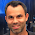Don't forget to also checkout my second blog containing articles to all other related ICT topics!!

Thursday, May 31, 2012

Python performance pitfalls (?) or issues (?)

I just want to warn you that Python has its glitches performance wise. Functional programming buzzwords are immutability and lazy evaluation (reduced memory footprint). But Python is doing something wrong here one would say. Check for yourself and I would love Python gurus to explain why my attempts to go with the lazy flow resulted in killing the performance.
```import cProfile

def vector_op(v1,v2,op):
return [op(x,y) for x,y in zip(v1,v2)]

v1 = range(1, 10000000)
v2 = range(1, 10000000)
cProfile.run('print sum(vector_op(v1,v2, lambda x,y: x + y))')
```

```99999990000000
10000046 function calls (10000040 primitive calls) in 59.064 seconds

ncalls  tottime  percall  cumtime  percall filename:lineno(function)
9999999   25.622    0.000   25.622    0.000 :1()
1   31.126   31.126   58.165   58.165 PythonApplication1.py:21(vector_op)
1    0.837    0.837    0.837    0.837 {sum}
1    1.417    1.417    1.417    1.417 {zip}
```

Let's not return an array but a generator expression.
```import cProfile

def vector_op_lazy(v1,v2,op):
return (op(x,y) for x,y in zip(v1,v2))

v1 = range(1, 10000000)
v2 = range(1, 10000000)
cProfile.run('print sum(vector_op_lazy(v1,v2, lambda x,y: x + y))')
```

What is really surprising is that it even takes more time now. So what are we doing still wrong?
```99999990000000
20000046 function calls (20000040 primitive calls) in 91.824 seconds

Ordered by: standard name

ncalls  tottime  percall  cumtime  percall filename:lineno(function)
9999999   26.132    0.000   26.132    0.000 :1()
10000000   46.346    0.000   72.478    0.000 PythonApplication1.py:25()
1   17.966   17.966   90.444   90.444 {sum}
1    1.378    1.378    1.378    1.378 {zip}
```

Let's also use xrange which is lazy evaluated and see how far that gets us.
```import cProfile

def vector_op_lazy(v1,v2,op):
return (op(x,y) for x,y in zip(v1,v2))

v1 = xrange(1, 10000000)
v2 = xrange(1, 10000000)
cProfile.run('print sum(vector_op_lazy(v1,v2, lambda x,y: x + y))')
```

```99999990000000
20000046 function calls (20000040 primitive calls) in 96.393 seconds

ncalls  tottime  percall  cumtime  percall filename:lineno(function)
9999999   27.329    0.000   27.329    0.000 :1()
10000000   48.976    0.000   76.305    0.000 PythonApplication1.py:27()
1   19.059   19.059   95.364   95.364 {sum}
1    1.028    1.028    1.028    1.028 {zip}
```

Will izip do us anygood in this case?
```import cProfile
from itertools import izip

def vector_op_lazy(v1,v2,op):
return (op(x,y) for x,y in izip(v1,v2))

v1 = xrange(1, 10000000)
v2 = xrange(1, 10000000)
cProfile.run('print sum(vector_op_lazy(v1,v2, lambda x,y: x + y))')
```

```99999990000000
20000045 function calls (20000039 primitive calls) in 91.346 seconds

ncalls  tottime  percall  cumtime  percall filename:lineno(function)
9999999   26.304    0.000   26.304    0.000 :1()
10000000   46.759    0.000   73.063    0.000 PythonApplication1.py:27()
1   18.280   18.280   91.343   91.343 {sum}
```

What about not using a lambda expression?
```import cProfile
from itertools import izip

def vector_op_lazy(v1,v2,op):
return (op(x,y) for x,y in izip(v1,v2))

return x + y

v1 = xrange(1, 10000000)
v2 = xrange(1, 10000000)
```

```99999990000000
20000045 function calls (20000039 primitive calls) in 89.603 seconds

ncalls  tottime  percall  cumtime  percall filename:lineno(function)
10000000   46.009    0.000   71.799    0.000 PythonApplication1.py:27()
9999999   25.790    0.000   25.790    0.000 PythonApplication1.py:29(op_add)
1   17.804   17.804   89.603   89.603 {sum}
```

Ok... last hope?! Let's inline the addition instead of calling operator function.
```import cProfile
from itertools import izip

def vector_op_lazy(v1,v2):
return (x + y for x,y in izip(v1,v2))

v1 = xrange(1, 10000000)
v2 = xrange(1, 10000000)

cProfile.run('print sum(vector_op_lazy(v1,v2))')
```

```99999990000000
10000046 function calls (10000040 primitive calls) in 45.078 seconds

ncalls  tottime  percall  cumtime  percall filename:lineno(function)
10000000   27.925    0.000   27.925    0.000 PythonApplication1.py:27()
1   17.153   17.153   45.077   45.077 {sum}
```

That seemed to help a lot, so beware of using too much abstraction when performance really matters. Not making the extra number of function calls reduces the execution time a lot. So now just for fun let us not return a generator expression but an array again.
```import cProfile
from itertools import izip

def vector_op(v1,v2):
return [x + y for x,y in zip(v1,v2)]

v1 = xrange(1, 10000000)
v2 = xrange(1, 10000000)
cProfile.run('print sum(vector_op(v1,v2))')
```

What the f@ck... The generator expression in combination with the sum function seems like total killing performance. Storing the result from the zip call in an array and next calling sum boosted performance.
```99999990000000
47 function calls (41 primitive calls) in 14.603 seconds
ncalls  tottime  percall  cumtime  percall filename:lineno(function)
1   12.678   12.678   13.733   13.733 PythonApplication1.py:25(vector_op)
1    0.810    0.810    0.810    0.810 {sum}
1    1.056    1.056    1.056    1.056 {zip}
```

This answer from stackoverflow is very useful to understand the outcome of this test. I conclude this article that it always makes good sense to profile your app and decide for yourself what is the best approach.

1.2.